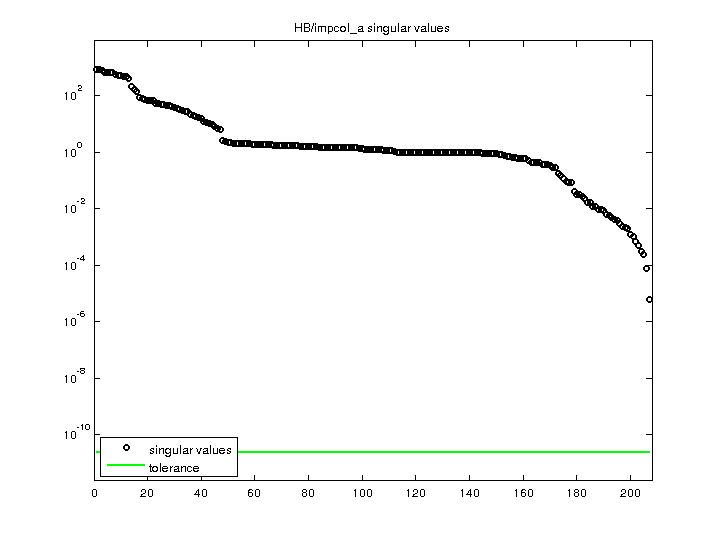Matrix: HB/impcol_a

Description: UNSYMMETRIC MATRIX - HEAT EXCHANGER NETWORK (CHEM ENG) ,1982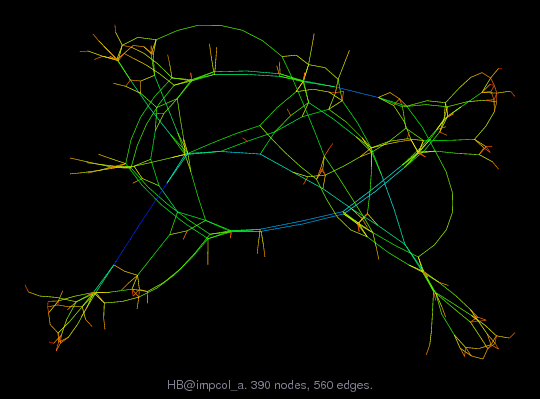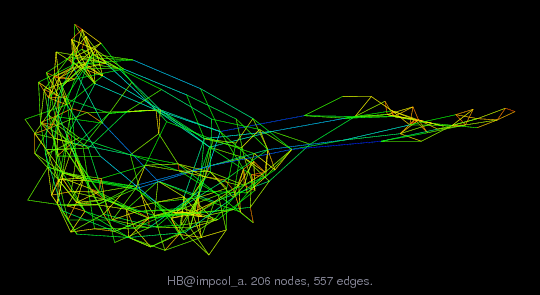(bipartite graph drawing) (graph drawing of A+A')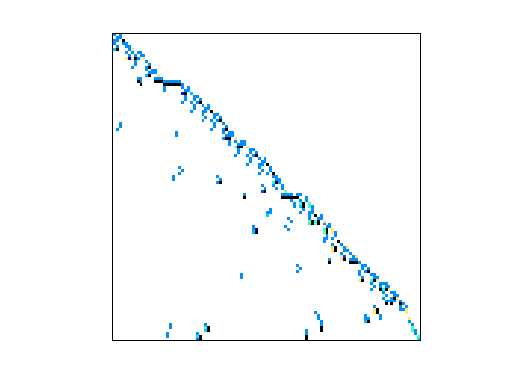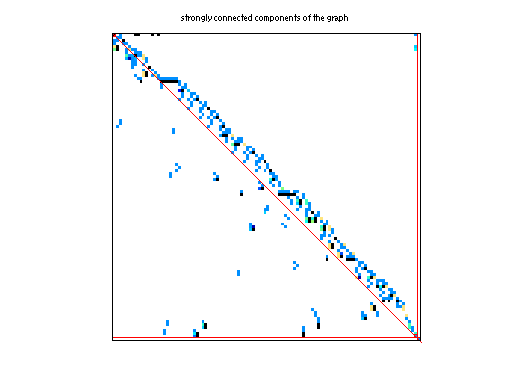• Matrix group: HB
• download as a MATLAB mat-file, file size: 4 KB. Use UFget(171) or UFget('HB/impcol_a') in MATLAB.

 Matrix properties number of rows 207 number of columns 207 nonzeros 572 structural full rank? yes structural rank 207 # of blocks from dmperm 164 # strongly connected comp. 4 explicit zero entries 0 nonzero pattern symmetry 2% numeric value symmetry 1% type real structure unsymmetric Cholesky candidate? no positive definite? no

 author D. Bogle editor I. Duff, R. Grimes, J. Lewis date 1982 kind chemical process simulation problem 2D/3D problem? no

 Ordering statistics: result nnz(chol(P*(A+A'+s*I)*P')) with AMD 2,721 Cholesky flop count 6.4e+04 nnz(L+U), no partial pivoting, with AMD 5,235 nnz(V) for QR, upper bound nnz(L) for LU, with COLAMD 573 nnz(R) for QR, upper bound nnz(U) for LU, with COLAMD 1,098

 SVD-based statistics: norm(A) 855.462 min(svd(A)) 6.32908e-06 cond(A) 1.35164e+08 rank(A) 207 sprank(A)-rank(A) 0 null space dimension 0 full numerical rank? yes

 singular values (MAT file): click here SVD method used: s = svd (full (A)) ; status: ok